Related Articles

# How to extract date from Excel file using Pandas?

• Difficulty Level : Basic
• Last Updated : 29 Dec, 2020

Prerequisite: Regular Expressions in Python

In this article, Let’s see how to extract date from the Excel file. Suppose our Excel file looks like below given image then we have to extract the date from the string and store it into a new Dataframe column.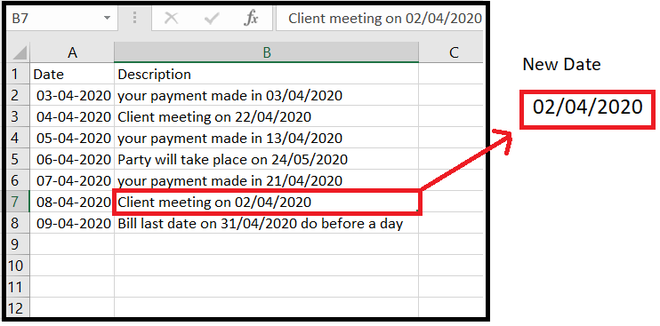date_sample_data.xlsx

Approach :

• Import required module.
• Import data from Excel file.
• Make an extra column for a new date.
• Set Index for searching.
• Define the pattern of date format.
• Search Date and assigning to the respective column in Dataframe.

Let’s see Step-By-Step-Implementation:

Step 1: Import the required module and read data from the Excel file.

## Python3

 `# import required module``import` `pandas as pd;``import` `re;`` ` `# Read excel file and store in to DataFrame``data ``=` `pd.read_excel(``"date_sample_data.xlsx"``);`` ` `print``(``"Original DataFrame"``)``data`

Output: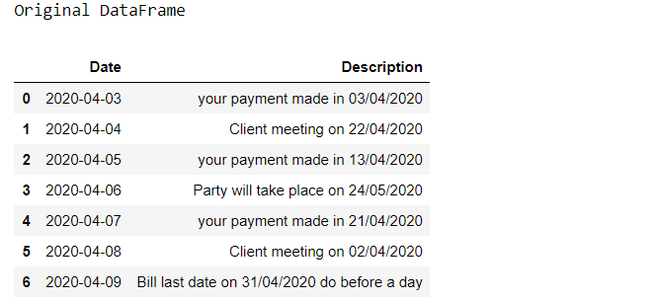Step 2: Make an extra column for a new date.

## Python3

 `# Create column for Date``data[``'new_Date'``]``=` `None``data`

Output: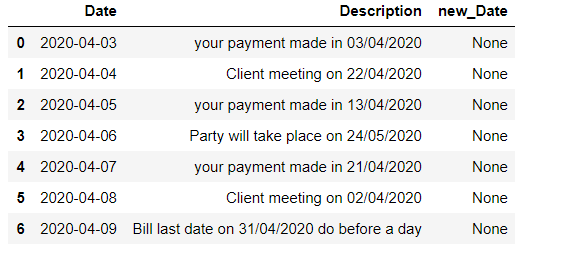Step 3: Set Index for searching.

## Python3

 `# set required index``index_set ``=` `data.columns.get_loc(``'Description'``)``index_date ``=` `data.columns.get_loc(``'new_Date'``)`` ` `print``(index_set, index_date)`

Output:

```1 2
```

Step 4: Defining the Pattern of the date format.

We need to create a Regular expression for date pattern in DD/MM/YY format. Use the [0-9] expression to find any character between the brackets that is a digit. Use escape sequence “\” for escaping “/” a special symbol and {2}, {4} is used to denote no of times a character belongs to the given string. So the expression become ‘[0-9]{2}\/[0-9]{2}\/[0-9]{4}’.

Example:

```02/04/2020
02 -----> [0 to 9] --> [0-9]
number of character inside the string {2} ( i.e DD)

04- ----> [0 to 9] --> [0-9]
number of character inside the string {2} ( i.e MM)

2020 -->[0 to 9] -->[0-9]
number of character inside the sting {4} ( i.e YYYY)
```

## Python3

 `# In DD/MM/YYYY``date_pattern ``=` `r``'([0-9]{2}\/[0-9]{2}\/[0-9]{4})'`

Step 5: Search Date and assigning to the respective column in Dataframe.

For searching the Date using regex in a string we are using re.search() function of re library.

## Python3

 `for` `row ``in` `range``(``0``, ``len``(data)):``    ``Date ``=` `re.search(date_pattern,data.iat[row,index_set]).group()``    ``data.iat[row, index_date] ``=` `Date``     ` `# show the Dataframe``data`

Output: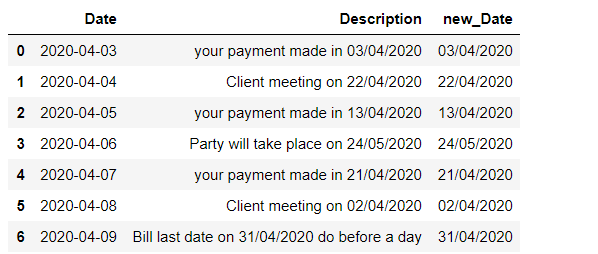Complete Code:

## Python3

 `# importing required module``import` `pandas as pd;``import` `re;`` ` `data ``=` `pd.read_excel(``"date_sample_data.xlsx"``);`` ` `print``(``"Original data : \n"``,``      ``data)`` ` `# Create column for Date``data[``'new_Date'``] ``=` `None`` ` `# set index``index_set ``=` `data.columns.get_loc(``'Description'``)``index_date ``=` `data.columns.get_loc(``'new_Date'``)``print``(index_set, index_date)`` ` `# define pattern for date``# in DD/MM/YYYY``date_pattern ``=` `r``'([0-9]{2}\/[0-9]{2}\/[0-9]{4})'`` ` `# searching pattern``# And storing in to DataFrame``for` `row ``in` `range``(``0``, ``len``(data)):``    ``Date ``=` `re.search(date_pattern, ``                     ``data.iat[row,index_set]).group()``    ``data.iat[row, index_date] ``=` `Date`` ` `# show the Dataframe``data`

Output: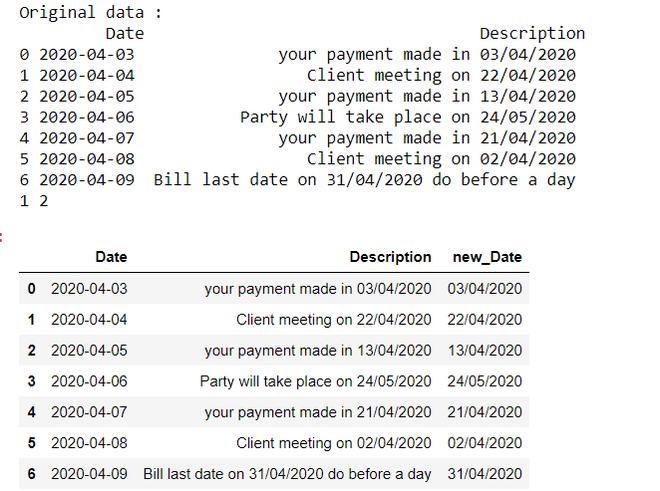Note: Before running this program, make sure you have already installed xlrd library in your Python environment.

Attention geek! Strengthen your foundations with the Python Programming Foundation Course and learn the basics.

To begin with, your interview preparations Enhance your Data Structures concepts with the Python DS Course. And to begin with your Machine Learning Journey, join the Machine Learning – Basic Level Course

My Personal Notes arrow_drop_up